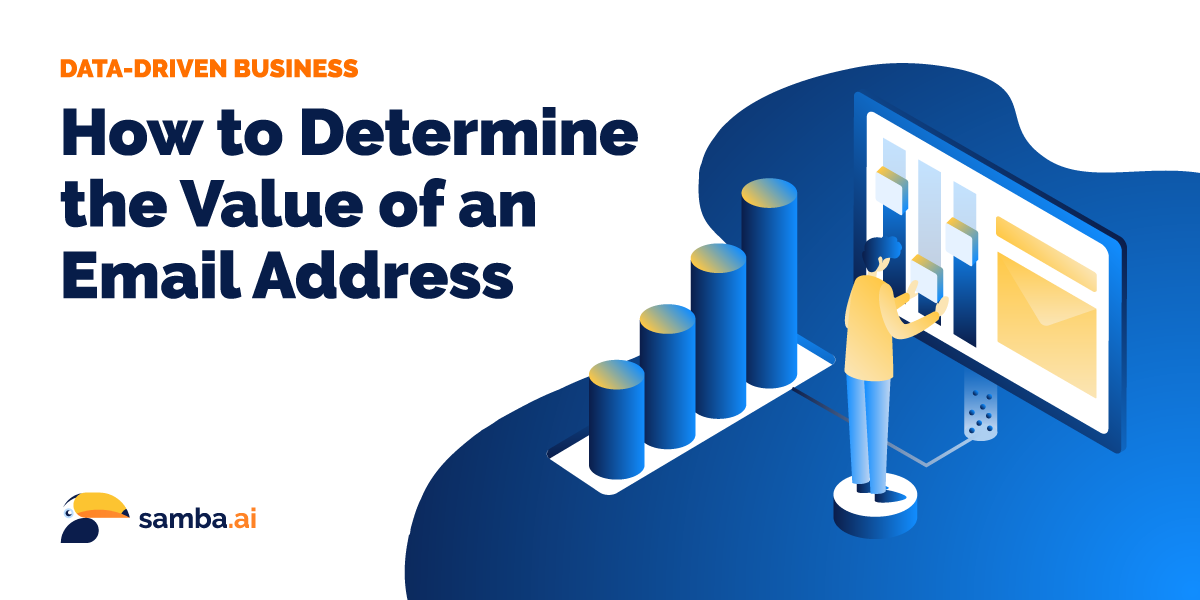22.12.2021
access_time
5
min

Since Ray Tomlinson brought the first email address to life over 45 years ago, digital communication technology has grown leaps and bounds and the technological finesse of the average person has grown along with it. Despite those advancements, the digital dinosaur that is email is still one of the most effective means of connecting with and sell to your customers and generating a massive return on your investment.

Spend a few minutes conducting a Google search on “email marketing statistics” and you’ll quickly discover objective fact after objective fact of how versatile and potent email marketing truly is. It’s that potential that makes email databases such an incredibly valuable part of any business’s marketing toolbox and filling up and maintaining that toolbox such an important responsibility.

Going a step further, understanding the actual value of an email address, and the subsequent means of doing so, helps you better fuel your email acquisition efforts, budget more effectively, create viable strategies, and quantify your marketing campaigns.

#### EMAIL ACQUISITION VALUE FORMULA

But how do you go about doing so? How do you attribute a value to an email address? To start, you have to dig into your typical email campaign statistics, which includes:

• Average Open Rate [denoted as “O”]: This is the percentage of opened emails
• Average Click-through Rate [denoted as “C”]: This is the percentage of clicked-through emails of those that have been opened
• Average Conversion Rate [denoted as “P”]: This is the percentage of conversions (according to your common attribution model) from clicked-through emails
• Average Order Value [denoted as “AOV”]
• Total Revenue generated by a campaign that targets all subscribers [denoted as “TR”]: Can be calculated using data from the preceding year:

or equivalently:

Please note: Only consider those subscribers that you regularly target.

• Average Profit Margin (see ) from revenue [denoted as “M”]
• Average Unsubscribe Rate [denoted as “U”]
• Number of Subscribers [denoted as “S”]
• Subscriber’s Lifespan [denoted as “T”]: This is the average number of newsletters received until someone unsubscribes

##### Step 1: Determine the gross profit contribution of each sent email

When you get down to it, the money you make on a marketing email is generally based on how often those subscribers (recipients) convert and the overall value you get when they do so. With that in mind, we can calculate profit contribution as such:

The factors involved in determining probability of conversion and the subsequent value of conversion include average open rate [O], average click rate [C], and average conversion rate [P]. Using the chain rule of probability theory () we get:

##### Step 2: Determine the subscriber’s lifespan

Next, you need to determine the longevity or churn of your customer in order to get an idea of how many campaigns they’ll be exposed to, on average, before they unsubscribe. The model of the unsubscribe process can be created as follows:

• 1st Campaign - You target all of your subscribers:
• 2nd Campaign - As a result of those who unsubscribe, you can only target:
• 3rd Campaign - You're only targeting the following subscribers:
• Nth campaign - You're only targeting the following subscribers:

For example, if your original number of subscribers is 100,000 and the unsubscribe rate is 10%, after two email campaigns you'll have only the following subscribers left:

After N campaigns, you’ve sent the following amount of emails:

which can be simplified using the sum of a geometric sequence :

Now, let’s consider a more substantial period in which we’re sending emails (perhaps upwards of a few hundred campaigns) and analyze the customers' unsubscribe process. Using the approximation of a geometric series  for large N and 0 %<U<100 %, the number of sent newsletters can be computed as follows:

Thus, we get an average subscriber lifespan as the total number of sent newsletters divided by the number of original subscribers:

For example, if your unsubscribe rate [U] is 10%, your subscriber’s lifespan is:

##### ‍Step 3: Determine expected gross profit

Finally, we can determine the expected gross profit per email address (i.e., the lifetime value of a single email subscriber) by multiplying the previously computed values:

Please note: We also could derive the same formula modeling the unsubscribe process by geometric distribution () with success probability equal to the unsubscribe rate as follows:

where X ~ Geo(U) is a random variable describing the number of trials until the recipient unsubscribes. A subscriber’s lifespan is then defined as the expected value of: